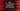# do...while loop in Dart with examples# do…while loop in dart :

do…while loop is similar to while loop. The only difference is that while loop checks the condition first and run a code block. But do…while loop runs the code block first and then it checks for the condition. If the condition is false, it stops.

If the condition is true always, it will keep running for an indefinite time. So, make sure that it becomes false at some point. You need to change its value inside the code block for that.

One more difference is that even if the condition is false for the first time, it will run for one time as it checks for the condition only after executing the code-block. while loop will not run if the condition is false because it checks for the condition before running the code-block.

## Syntax of do…while loop :

The syntax of do…while loop is as below :

``````do{
// code block to execute
} while (condition);
``````

Note that the semicolon is required at the end of do…while loop. It is not required for while and for loops.

## Example 1: Print from 1 to n using do…while in dart :

The below program will print all numbers from 1 to n using a do…while loop :

``````import 'dart:io';

main() {
var i = 1;
var n = 10;
do {
print("i : \$i");
} while (i++ < n);
}
``````

It will print the below output :

``````i : 1
i : 2
i : 3
i : 4
i : 5
i : 6
i : 7
i : 8
i : 9
i : 10
``````

If you use one while loop, the same program will look as like below :

``````import 'dart:io';

main() {
var i = 0;
var n = 10;
while (i++ < n) {
print("i : \$i");
}
}
``````

Noticed the difference ? We don’t have one ; for the while loop and the initial value of i is 0. This is because the value of i is incremented first before running the code block for the while loop.

## Example 2: Find the factorial of a number using do-while :

``````main() {
var n = 10;
var factorial = 1;
do {
print("multiplying \$factorial with \$n");
factorial *= n;
} while (n-- > 2);

print("factorial = \$factorial");
}
``````

It will calculate the factorial of 10. Output :

``````multiplying 1 with 10
multiplying 10 with 9
multiplying 90 with 8
multiplying 720 with 7
multiplying 5040 with 6
multiplying 30240 with 5
multiplying 151200 with 4
multiplying 604800 with 3
multiplying 1814400 with 2
factorial = 3628800
``````